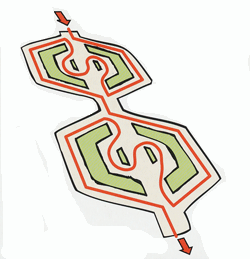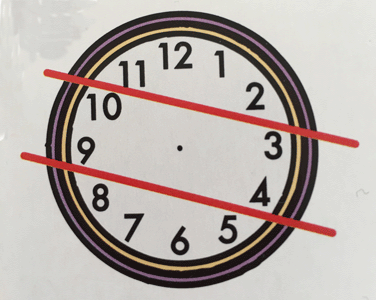Select Page

August 2016

Lots of Working Memory practice for you this month.

Here are the answers to the August 2016 Brain Practice:

Park Puzzle

Here is Oscar’s route.How Many Exchanges were there?

Each of the 20 businessmen gave a coin to all of the others (apart from himself) so that country’s coin is given to 19 people. Therefore the total number of ‘giving’s was 20 x 19 = 380.

Since one exchange is two ‘givings’, the total number of exchanges is 380 divided by 2 – 190.

A Timely ChallengeUse the two straight lines to divide the clock-face into threes. Then add together the digits contained in each segment.

Did you find a word with 3 sets of double letters, all following each other?

We found the word BOOKKEEPER. Did you find any others?